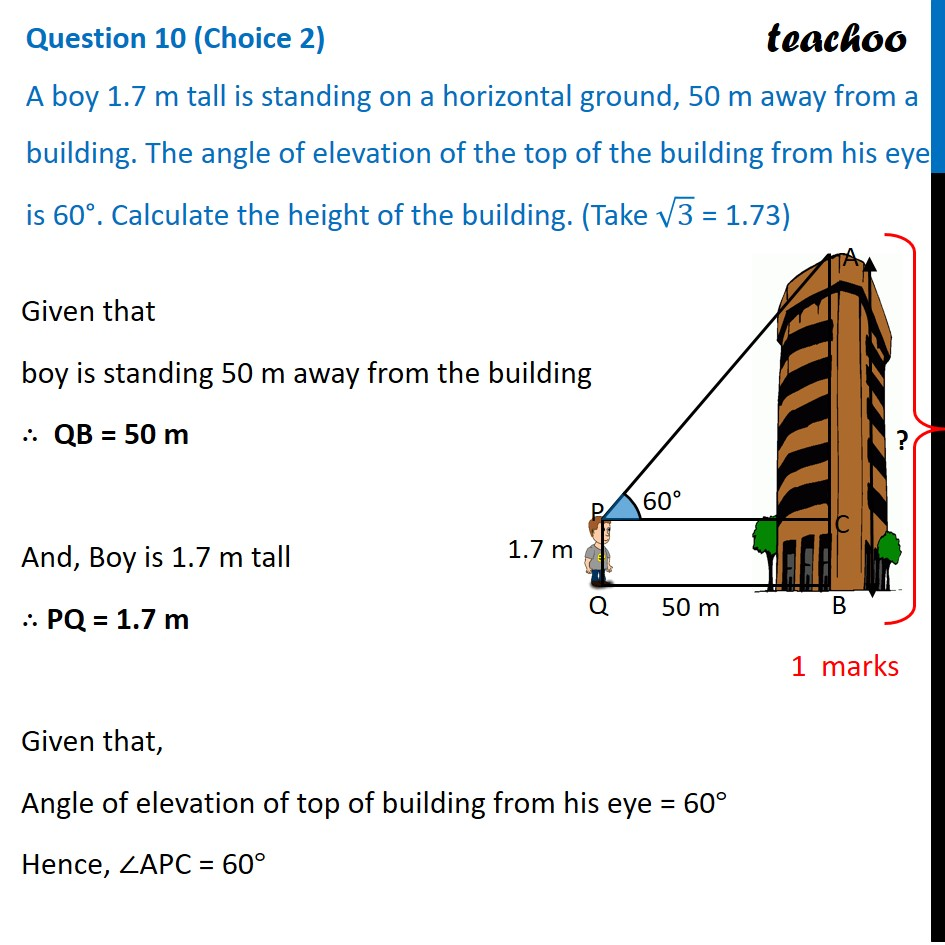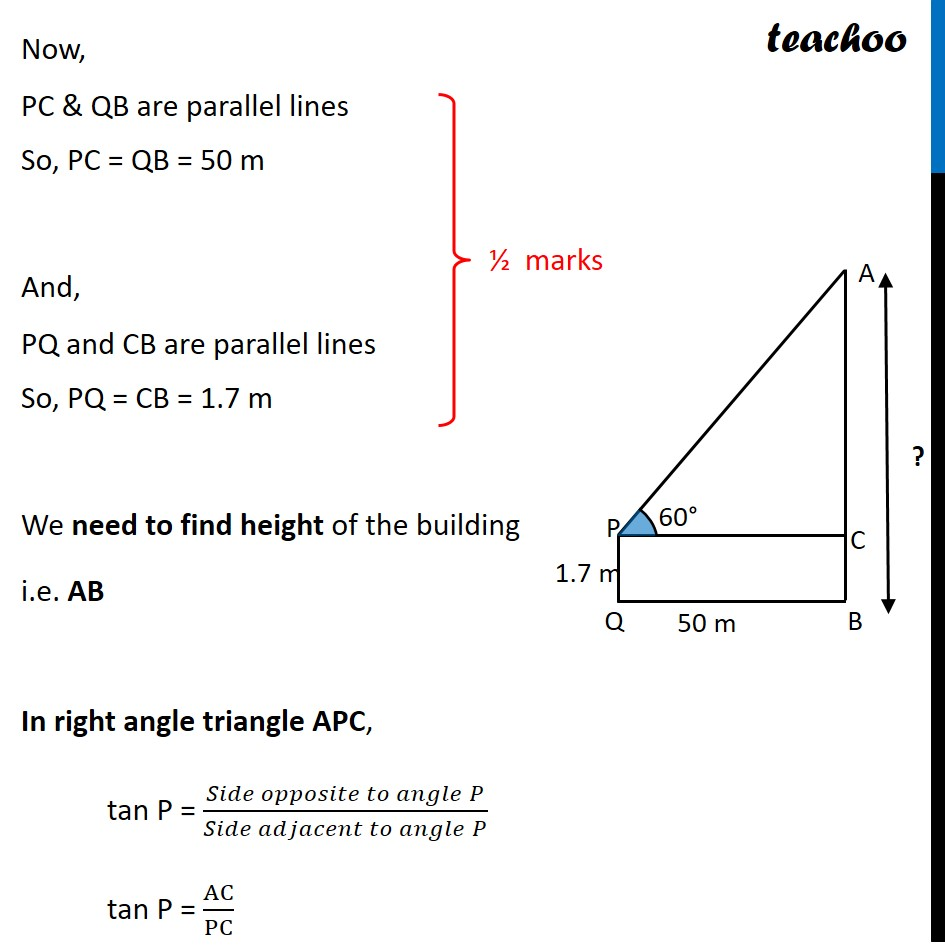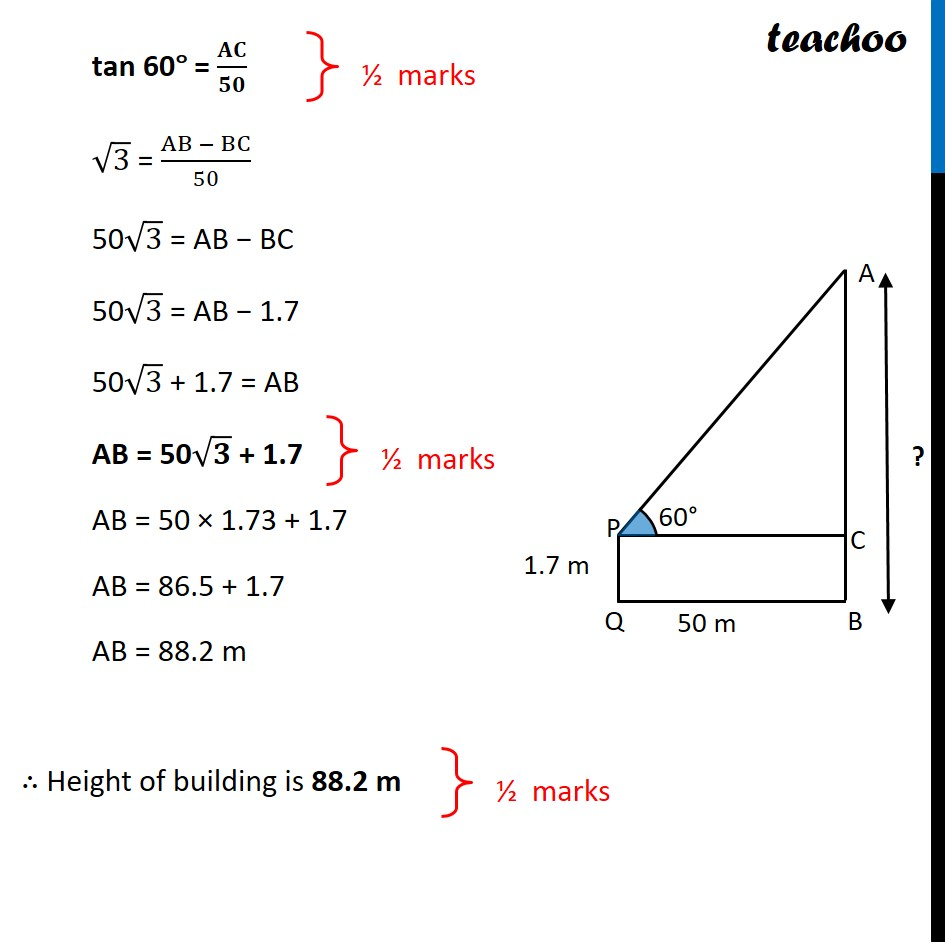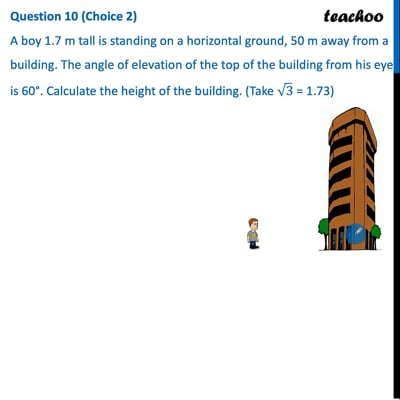CBSE Class 10 Sample Paper for 2022 Boards - Maths Standard [Term 2]

Class 10
Solutions of Sample Papers for Class 10 Boards

## A boy 1.7 m tall is standing on a horizontal ground, 50 m away from a building. The angle of elevation of the top of the building from his eye is 60°. Calculate the height of the building. (Take √3 = 1.73)This video is only available for Teachoo black users

### Transcript

Question 10 (Choice 2) A boy 1.7 m tall is standing on a horizontal ground, 50 m away from a building. The angle of elevation of the top of the building from his eye is 60°. Calculate the height of the building. (Take √3 = 1.73) Given that boy is standing 50 m away from the building ∴ QB = 50 m And, Boy is 1.7 m tall ∴ PQ = 1.7 m Given that, Angle of elevation of top of building from his eye = 60° Hence, ∠APC = 60° Now, PC & QB are parallel lines So, PC = QB = 50 m And, PQ and CB are parallel lines So, PQ = CB = 1.7 m We need to find height of the building i.e. AB In right angle triangle APC, tan P = (𝑆𝑖𝑑𝑒 𝑜𝑝𝑝𝑜𝑠𝑖𝑡𝑒 𝑡𝑜 𝑎𝑛𝑔𝑙𝑒" " 𝑃)/(𝑆𝑖𝑑𝑒 𝑎𝑑𝑗𝑎𝑐𝑒𝑛𝑡 𝑡𝑜 𝑎𝑛𝑔𝑙𝑒" " 𝑃) tan P = AC/PC tan 60° = 𝐀𝐂/𝟓𝟎 √3 = (AB − BC)/50 50√3 = AB − BC 50√3 = AB − 1.7 50√3 + 1.7 = AB AB = 50√𝟑 + 1.7 AB = 50 × 1.73 + 1.7 AB = 86.5 + 1.7 AB = 88.2 m ∴ Height of building is 88.2 m